Coding is an art! Anyone can draw, but only those who draw with the elegant have their paintings valued. Similarly any programmer can code, but a Good Program is an artistic job. I see lot of people do code, but only few actually write Good code. This means they have understood the elegance of the language and knows how to use it.

### Big Code - Good Code

Big Code, a Good Code? Sounds awkward? A Good code I mean here is a code which runs quicker than other solutions for the same problem.

if T(Sol1) < T(Sol2) Then, Solution 1 is a Good code., Where T is Time function , measures Time Taken

( though there are various other constraints involved, for time being let not discuss them )

Hardcore programmers are always tries to write short programs. They couple few lines of code into one and show that they have done a great coding.  But, things are not always the way it looks. Short is sweet, but not always !

Look into this code, 3 solutions for one simple problem.

Problem: Find the greatest of two numbers a, b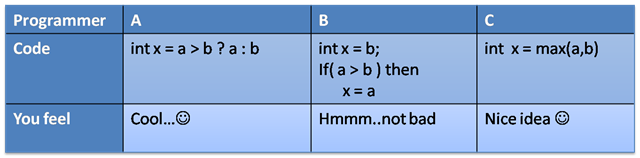### Million Dollar Question

Which code runs faster? If you have chosen the A’s code as faster. You have correctly made a wrong decision.

The fastest program is the B’s program. So, 3 lines of code, still fastest code? Ok.

In the A’s code,  consider if a < b, two operations should be performed.

• Compare 'a' , 'b'
• Branch to Else if 'a' < 'b'
• Return the expression 'b'

But in B’s solution, we have already assigned a value to ‘x’. Only job is to compare and Assign. So the second operation is saved.

In such a small code, you won’t find a big difference. But think of a macro system with MLOC ( Million Lines of Code ).

I will explain you by solving a bit complex problem, come on join me.

Problem: Given number ‘N’ , split into ‘k’ integers, such that N = k1 + k2 + k3 +…kn and k1 * k2 * k3 is maximum.

You can try various solutions. One simple solution is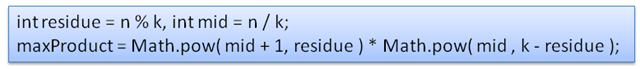Great code, you would be proud to write one line solution of such great problem. But wait, is your code is faster to run for N > 10^10, K > 10^5?

Consider the optimize solution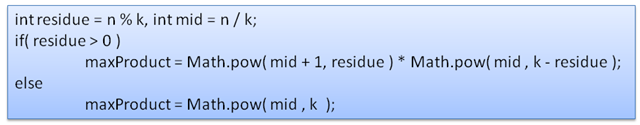More optimised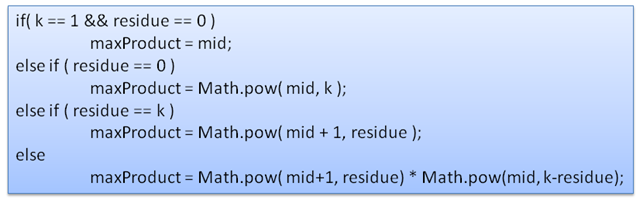The last code works much faster than any other. Try looping it for million times with various values of ‘N & k ‘. and measure the time it takes to complete the million time execution.

### How to write a speedy code?

So, this is yet another million dollar question. Take a look at the above three solutions. You will find a resemblance.

Solution - 1 is a generalized form of other two. Solution - 3 is more detailed form of Solution - 1.

So, what is been detailed in the Solution 3. If you look at more closely, you will find that Solution-3 has checks for ‘Boundary Conditions’.

Boundary Conditions are very important scenarios in any Logical System. You prove that system works perfect for boundary conditions, you prove the system to work for any values between it. Similar to Mathematical Induction. So, this applies for programming too.

If you are able to identify the boundary conditions, and make the appropriate formula for those conditions, then you will skip unnecessary computations.

We will look what have save the computational cycles.

Lets assume, the Input are N=10, K = 2

Using Solution-1:

residue = 0, mid = 5

maxProduct = Math.pow(5+1,0) * Math.pow( 5 ,(2-0) ) = 25

Operations performed:

Note: costs are just for calculation, not exact one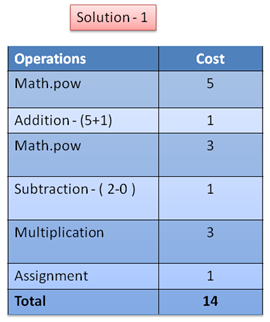• Math.pow
• Math.pow
• Subtraction - ( 2-0 )
• Multiplication

lets assume the cost for each operation is

Using Solution-3:

The code will branch out to the 1st if condition ( residue == 0 )

maxProduct = Math.pow( 5, 2) = 25

Operations performedSo, you have saved more than 55% of the computational cycles by covering up the boundary conditions.

So, Why you need a Speedy code?

Everyone in life loves speed. Speed bike, Speed cars ( that’s why you would love F1 ), A speedy 2 GB - Core 2 Duo Powered Machine.

Because Speed makes you feel Good !! So does a Speedy Code will be a Good code.

But, to achieve something you have to compromise with other factors, like I mentioned earlier.

If you need a 2 GB - Core 2 Duo Powered Machine, you should not consider the Money factor.

Similarly while you code, there are lot of other factors like

• Memory Management
• Security
• Transaction Isolations etc.,

This is just a introduction, will start covering up other topics soon.

Posted on: